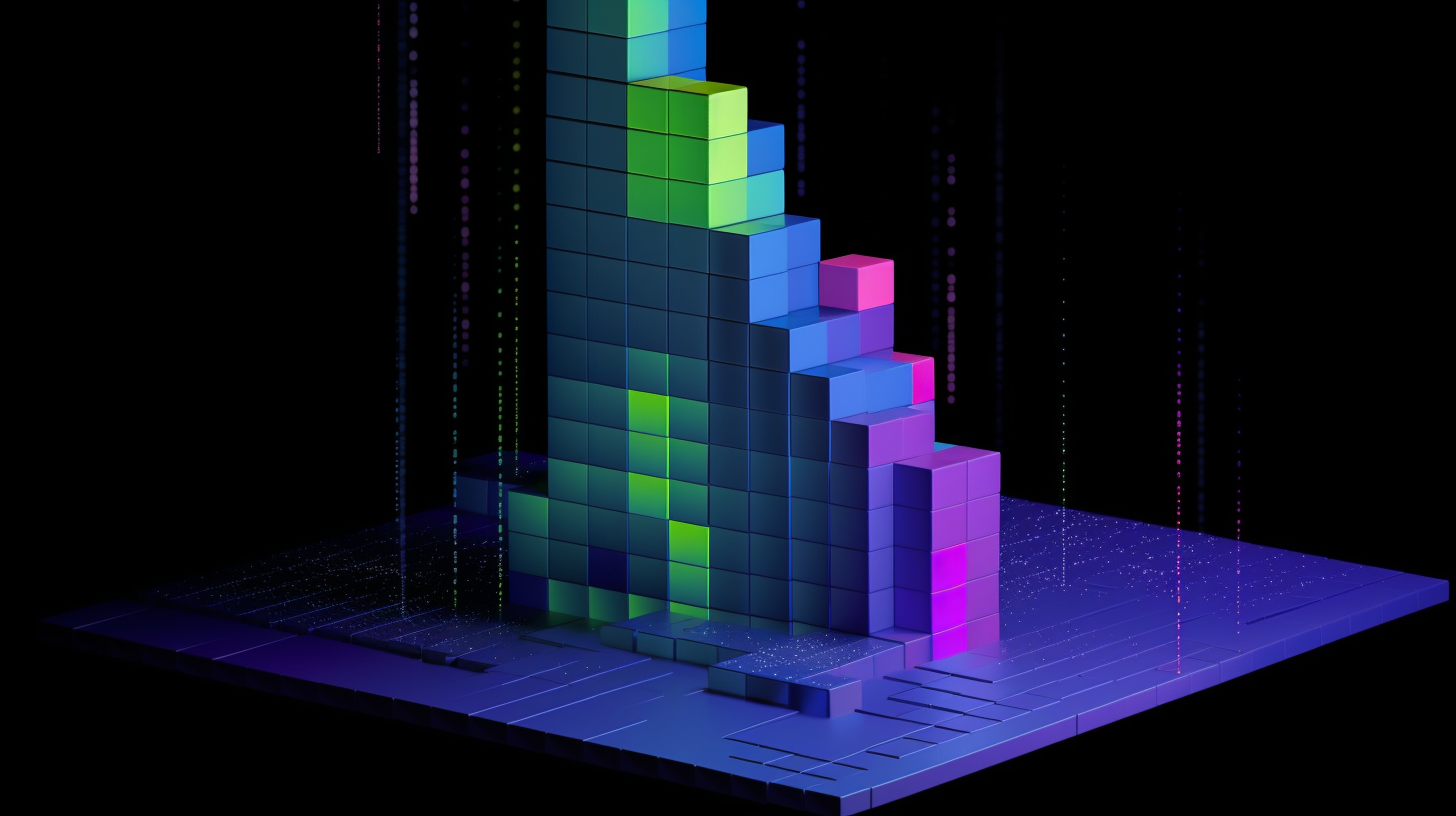# Data Analysis & Visualization Fluency

Course

Learn about the data analysis process, key steps in data wrangling, basic data types, summary statistics, how to design good data visualizations, common types of charts and plots, and how to tell a story with your visuals.

Learn about the data analysis process, key steps in data wrangling, basic data types, summary statistics, how to design good data visualizations, common types of charts and plots, and how to tell a story with your visuals.

5 hours

Completion Certificate

Last Updated September 8, 2022

## Course Lessons

Lesson 1

What is Data Analysis?

In this lesson, you will learn about the basics of data analysis and visualization, such as the data analysis process and when to use data visualization.

Lesson 2

Data Wrangling

In this lesson, you will learn about the key steps in data wrangling, which gets your data ready for analysis and exploration.

Lesson 3

Descriptive Statistics

In this lesson, you will learn about the basics of data analysis such as data types and summary statistics.

Lesson 4

Data Visualization

In this lesson, you will learn about how to design good data visualizations, common types of charts and plots, and how to tell a story with your visuals.Get Started Today

Data Analysis & Visualization Fluency